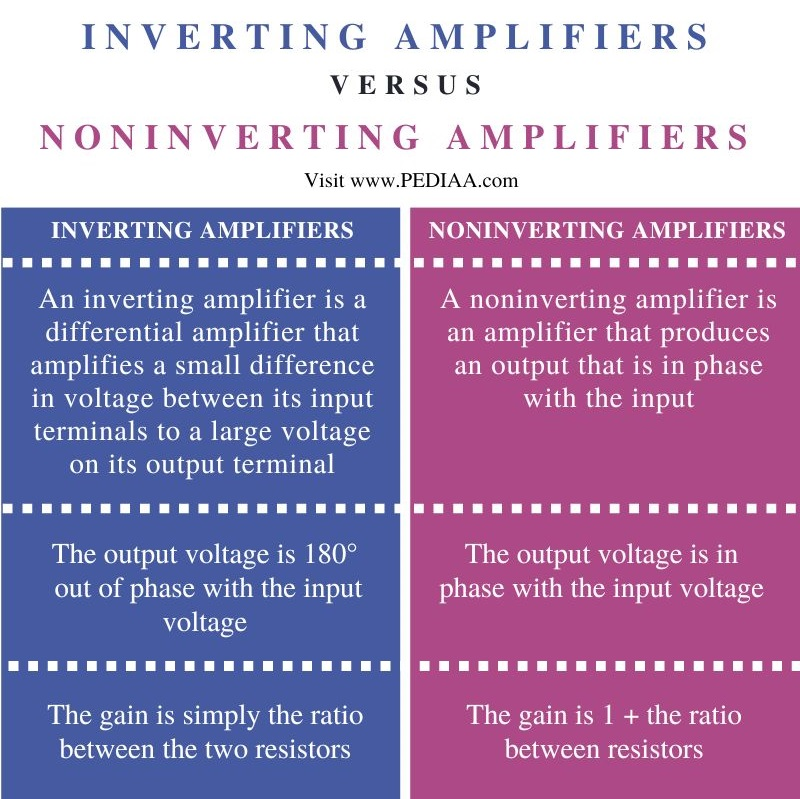# Difference Between Inverting and Noninverting Amplifier

The main difference between inverting and noninverting amplifier is that an inverting amplifier produces an output that is 180o out of phase with the input, whereas a noninverting amplifier produces an output that is in phase with the input.

Operational amplifiers can be arranged in two fundamental configurations: inverting and noninverting amplifiers. An inverting amplifier is a differential amplifier that amplifies a small difference in voltage between its input terminals to a large voltage on its output terminal. On the other hand, a noninverting amplifier is an amplifier that produces an output that is in phase with the input.

### Key Areas Covered

1. What is an Inverting Amplifier
– Definition, Features
2. What is a Noninverting Amplifier
– Definition, Features
3. Difference Between Inverting and Noninverting Amplifier
– Comparison of Key Differences

### Key Terms

Inverting Amplifier, Noninverting Amplifier, Operational Amplifiers## What is an Inverting Amplifier

An inverting amplifier is a differential amplifier that amplifies a small difference in voltage between its input terminals to a large voltage on its output terminal. The output voltage is at 180o out of phase compared to the input voltage. The setup of an inverting amplifier is shown below.Figure 1: An Operational Amplifier

For amplifiers, the output voltage$V_{out}$ is given in terms of the two input voltages$V^+$ and$V_-$ as:$V_{out}=A\left( V_+-V_-\right)$

where$A$ is the gain of the operational amplifier. Since$V_+$ is connected to Earth,$V_+=0$. The point marked$X$ is virtual Earth so the potential at this point is almost 0 V. No current passes through this point. Therefore the current that flows through the resistor$R_2$ is the same as the current$I$ that flows through the resistor$R_1$.

If the potential difference across$R_1$ is$V_1$, then,$I=\frac{V_1}{R_1}=\frac{V_{in}-0}{R_1}=\frac{V_{in}}{R_1}$

Similarly for$R_2$$I=\frac{V_2}{R_2}=\frac{0-V_{out}}{R_2}=-\frac{V_{out}}{R_2}$

Then the gain$A$ can be expressed as:$A=\frac{V_{out}}{V_{in}}=\frac{-IR_2}{IR_1}$$A=-\frac{R_2}{R_1}$

The negative sign here indicates that the output is at a 180o phase difference with the input.

## What is a Noninverting Amplifier

A noninverting amplifier is an amplifier that produces an output that is in phase with the input. The setup of a non-inverting amplifier is shown below:Figure 2: A Noninverting Amplifier

In the noninverting amplifier,$V_{in}=V_+$. The gain in a noninverting amplifier is quite large, so the difference between$V_+$ and$V_-$ is negligible. So we can say that$V_-\approx V_+ \left( =V_{in}\right)$. Using the idea of a potential divider, we can write$V_-=V_{out}\times \frac{R_2}{R_1+R_2}$. Equating the two expressions for$V_-$, we have:$V_{in}=V_{out}\times \frac{R_2}{R_1+R_2}$. Then, we can write the gain as:$A=\frac{V_{out}}{V_{in}}=\frac{R_1+R_2}{R_2}$. Dividing both the numerator and the denominator by$R_2$, we get:$A=1+\frac{R_1}{R_2}$

## Difference Between Inverting and Noninverting Amplifiers

### Definition

An inverting amplifier is a differential amplifier that amplifies a small difference in voltage between its input terminals to a large voltage on its output terminal. On the other hand, a noninverting amplifier is an amplifier that produces an output that is in phase with the input.

### Phase Difference

In an inverting amplifier, the output voltage is 180o out of phase with the input voltage, but in a noninverting amplifier, the output voltage is in phase with the input voltage.

### Amplifier Gain

For an inverting amplifier, the gain is simply the ratio between the two resistors, while for a noninverting amplifier, the gain is 1 + the ratio between resistors.

## Conclusion

Operational amplifiers can be arranged in two fundamental configurations: inverting and noninverting amplifiers. The main difference between inverting and noninverting amplifier is that an inverting amplifier produces an output that is 180o out of phase with the input, whereas a noninverting amplifier produces an output that is in phase with the input.

##### Reference:

1. “Inverting Operational Amplifier.” Electronics Tutorial.